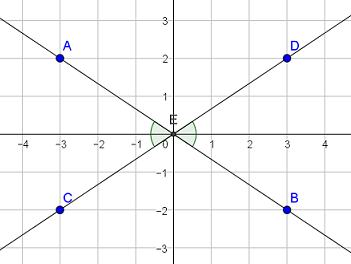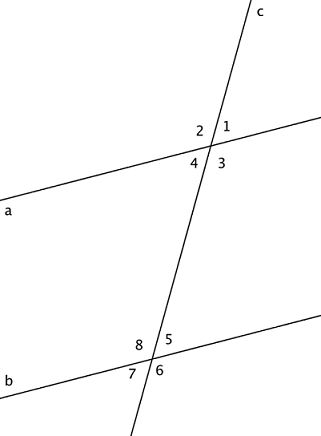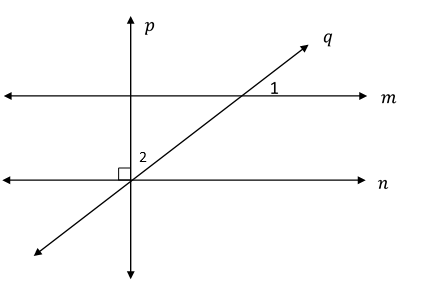Match Fishtank is now Fishtank Learning!

# Transformations and Angle Relationships

## Objective

Define and identify corresponding angles in parallel line diagrams. Review vertical, supplementary, and complementary angle relationships.

## Common Core Standards

### Core Standards

?

• 8.G.A.2 — Understand that a two-dimensional figure is congruent to another if the second can be obtained from the first by a sequence of rotations, reflections, and translations; given two congruent figures, describe a sequence that exhibits the congruence between them.

• 8.G.A.5 — Use informal arguments to establish facts about the angle sum and exterior angle of triangles, about the angles created when parallel lines are cut by a transversal, and the angle-angle criterion for similarity of triangles. For example, arrange three copies of the same triangle so that the sum of the three angles appears to form a line, and give an argument in terms of transversals why this is so.

?

• 7.G.B.5

## Criteria for Success

?

1. Know angle relationship facts about complementary, supplementary, and vertical angles.
2. Prove that vertical angles are congruent using a reflection.
3. Identify corresponding angles in parallel line diagrams.
4. Prove that corresponding angles in parallel line diagrams are congruent using translations.

## Tips for Teachers

?

• Students may need to review 7.G.5 before they can fully access this lesson and the lessons to follow in this section. Anchor Problem #1 reviews vertical and supplementary angles, but students may need additional review of other angle relationships from seventh grade. These concepts and vocabulary will support students' problem-solving abilities as they encounter more challenging and comlex angle relationship diagrams.
• The following materials are useful for this lesson: patty (transparency) paper, protractors, and graph paper.

#### Remote Learning Guidance

If you need to adapt or shorten this lesson for remote learning, we suggest prioritizing Anchor Problem 1 (benefits from discussion) . Find more guidance on adapting our math curriculum for remote learning here.

#### Fishtank Plus

• Problem Set
• Student Handout Editor
• Vocabulary Package

## Anchor Problems

?

### Problem 1

Two lines intersect at the origin, as shown in the coordinate plane below.1. What is the relationships between ${{\angle AEC}}$ and ${\angle DEB}$?
2. What is the relationship between ${{\angle AEC}}$ and ${\angle AED}$?

### Problem 2

In the diagram below, lines $a$ and $b$ are parallel. Line $c$ is a transversal that cuts through the parallel lines.1. Name four pairs of congruent vertical angles.
2. $\angle 2$ and $\angle 8$ are congruent. How can you prove this?
3. Name three other pairs of corresponding angles.

## Problem Set

?The following resources include problems and activities aligned to the objective of the lesson that can be used to create your own problem set.

• Examples where students find all angles that are equivalent to a given angle measure in a parallel line diagram and explain why (This will include alt interior/exterior angles, but students do not need to name these yet, as this comes in the next lesson).

?

In the diagram below, lines $m$ and $n$ are parallel. Line $p$ is a transversal that is perpendicular to lines $m$ and $n$. Line $q$ is another transversal.If $\angle 1$ is ${41°}$, then what is the measure of $\angle 2$? Explain how you determined your answer using appropriate vocabulary.

?